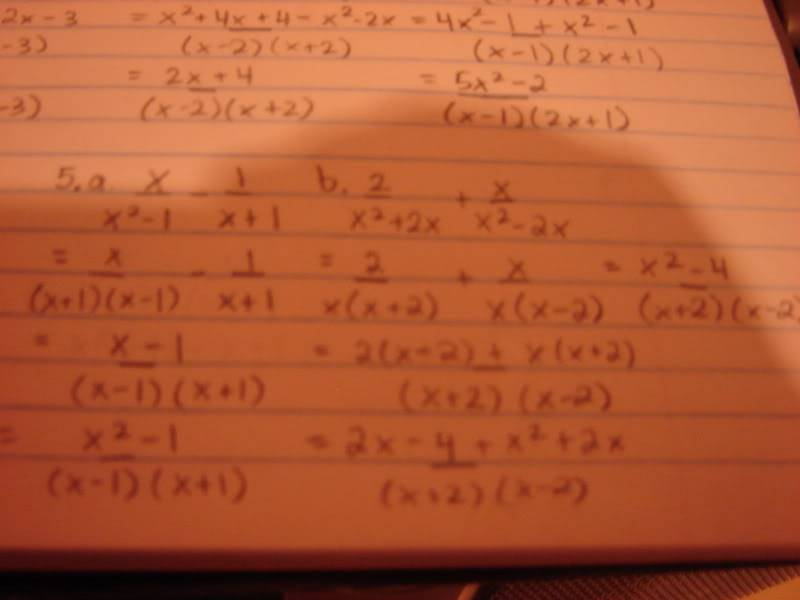# Adding and subtracting Rational Expressions

• caprija

#### caprija

I'm stuck on how to do this problem

My attempt:the answer to the second one is 3x + 2/(x+2)(x-2)

Can someone point out my mistake?

When you said that:

$$\frac{2}{x(x+2)} + \frac{x}{x(x-2)} = \frac{x^2-4}{(x+2))(x-2)}$$

That is incorrect (take x = 1 and you get -1/3 = 1).

Also, just as a style note: you should write the fraction bar so that it extends over both the top and the bottom. (does that make sense?) For example, on your last line, you have a little bar below the 4, you should instead have a bar going from the start of the first 2x to the end of the last 2x. Unless of course you meant to write:
$$2x - \frac{4}{(x+2)(x-2)} + x^2 + 2x$$

edit... also the "answer" you wrote is not correct.

Last edited:
Alright, Thank you.